MATLAB Function Referencebesselk

Modified Bessel function of the second kind

Syntax

• ```K = besselk(nu,Z)
K = besselk(nu,Z,1)
[K,ierr] = besselk(...)
```

Definitions

The differential equation

•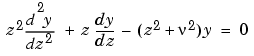where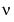is a real constant, is called the modified Bessel's equation, and its solutions are known as modified Bessel functions.

A solution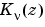of the second kind can be expressed as

•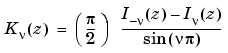where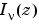and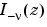form a fundamental set of solutions of the modified Bessel's equation for noninteger•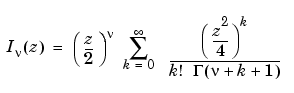and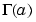is the gamma function.is independent of.can be computed using `besseli`.

Description

```K = besselk(nu,Z) ``` computes the modified Bessel function of the second kind,, for each element of the array `Z`. The order `nu` need not be an integer, but must be real. The argument `Z` can be complex. The result is real where `Z` is positive.

If `nu` and `Z` are arrays of the same size, the result is also that size. If either input is a scalar, it is expanded to the other input's size. If one input is a row vector and the other is a column vector, the result is a two-dimensional table of function values.

```K = besselk(nu,Z,1) ``` computes `besselk(nu,Z).*exp(Z)`.

```[K,ierr] = besselk(...) ``` also returns completion flags in an array the same size as `K`.

 ierr Description `0` `besselk` successfully computed the modified Bessel function for this element. `1` Illegal arguments. `2` Overflow. Returns `Inf`. `3` Some loss of accuracy in argument reduction. `4` Unacceptable loss of accuracy, `Z` or `nu` too large. `5` No convergence. Returns `NaN`.

Examples

Example 1.

• ```format long
z = (0:0.2:1)';

besselk(1,z)

ans =
Inf
4.77597254322047
2.18435442473269
1.30283493976350
0.86178163447218
0.60190723019723
```

Example 2. `besselk(3:9,(0:.2:10)',1)` generates part of the table on page 424 of  Abramowitz and Stegun, Handbook of Mathematical Functions.

Algorithm

The `besselk` function uses a Fortran MEX-file to call a library developed by D. E. Amos  .

See Also

`airy`, `besselh`, `besseli`, `besselj`, `bessely`

References

  Abramowitz, M. and I.A. Stegun, Handbook of Mathematical Functions, National Bureau of Standards, Applied Math. Series #55, Dover Publications, 1965, sections 9.1.1, 9.1.89 and 9.12, formulas 9.1.10 and 9.2.5.

  Carrier, Krook, and Pearson, Functions of a Complex Variable: Theory and Technique, Hod Books, 1983, section 5.5.

  Amos, D. E., "A Subroutine Package for Bessel Functions of a Complex Argument and Nonnegative Order," Sandia National Laboratory Report, SAND85-1018, May, 1985.

  Amos, D. E., "A Portable Package for Bessel Functions of a Complex Argument and Nonnegative Order," Trans. Math. Software, 1986.

© 1994-2005 The MathWorks, Inc.